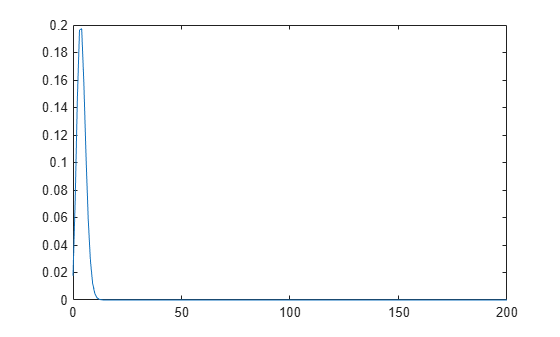# binopdf

Binomial probability density function

## Syntax

``y = binopdf(x,n,p)``

## Description

example

````y = binopdf(x,n,p)` computes the binomial probability density function at each of the values in `x` using the corresponding number of trials in `n` and probability of success for each trial in `p`.`x`, `n`, and `p` can be vectors, matrices, or multidimensional arrays of the same size. Alternatively, one or more arguments can be scalars. The `binopdf` function expands scalar inputs to constant arrays with the same dimensions as the other inputs.```

## Examples

collapse all

Compute and plot the binomial probability density function for the specified range of integer values, number of trials, and probability of success for each trial.

In one day, a quality assurance inspector tests 200 circuit boards. 2% of the boards have defects. Compute the probability that the inspector will find no defective boards on any given day.

`binopdf(0,200,0.02)`
```ans = 0.0176 ```

Compute the binomial probability density function values at each value from 0 to 200. These values correspond to the probabilities that the inspector will find 0, 1, 2, ..., 200 defective boards on any given day.

```defects = 0:200; y = binopdf(defects,200,.02);```

Plot the resulting binomial probability values.

`plot(defects,y)`Compute the most likely number of defective boards that the inspector finds in a day.

```[x,i] = max(y); defects(i)```
```ans = 4 ```

## Input Arguments

collapse all

Values at which to evaluate the binomial pdf, specified as an integer or an array of integers. All values of `x` must belong to the interval ```[0 n]```, where `n` is the number of trials.

Example: `[0,1,3,4]`

Data Types: `single` | `double`

Number of trials, specified as a positive integer or an array of positive integers.

Example: `[10,20,50,100]`

Data Types: `single` | `double`

Probability of success for each trial, specified as a scalar value or an array of scalar values. All values of `p` must belong to the interval `[0 1]`.

Example: `[0.01,0.1,0.5,0.7]`

Data Types: `single` | `double`

## Output Arguments

collapse all

Binomial pdf values, returned as a scalar value or array of scalar values. Each element in `y` is the binomial pdf value of the distribution evaluated at the corresponding element in `x`.

Data Types: `single` | `double`

collapse all

### Binomial Probability Density Function

The binomial probability density function lets you obtain the probability of observing exactly x successes in n trials, with the probability p of success on a single trial.

The binomial probability density function for a given value x and given pair of parameters n and p is

`$y=f\left(x|n,p\right)=\left(\begin{array}{c}n\\ x\end{array}\right){p}^{x}{q}^{\left(n-x\right)}{I}_{\left(0,1,...,n\right)}\left(x\right)$`

where q = 1 – p. The resulting value y is the probability of observing exactly x successes in n independent trials, where the probability of success in any given trial is p. The indicator function I(0,1,...,n)(x) ensures that x only adopts values of 0, 1, ..., n.

## Alternative Functionality

• `binopdf` is a function specific to binomial distribution. Statistics and Machine Learning Toolbox™ also offers the generic function `pdf`, which supports various probability distributions. To use `pdf`, specify the probability distribution name and its parameters. Alternatively, create a `BinomialDistribution` probability distribution object and pass the object as an input argument. Note that the distribution-specific function `binopdf` is faster than the generic function `pdf`.

• Use the Probability Distribution Function app to create an interactive plot of the cumulative distribution function (cdf) or probability density function (pdf) for a probability distribution.